So the first thing I have learned from this exercise is that I am really bad at coding. More often than not I did not create what I was trying to create. But after watching some of Patrick Conner’s YouTube videos I started playing around just to see if I could do any of the things he was demonstrating.

This was my first little “project”. Basically I just created a cube and then used translate to add identical cubes beside it, which created a little stair step.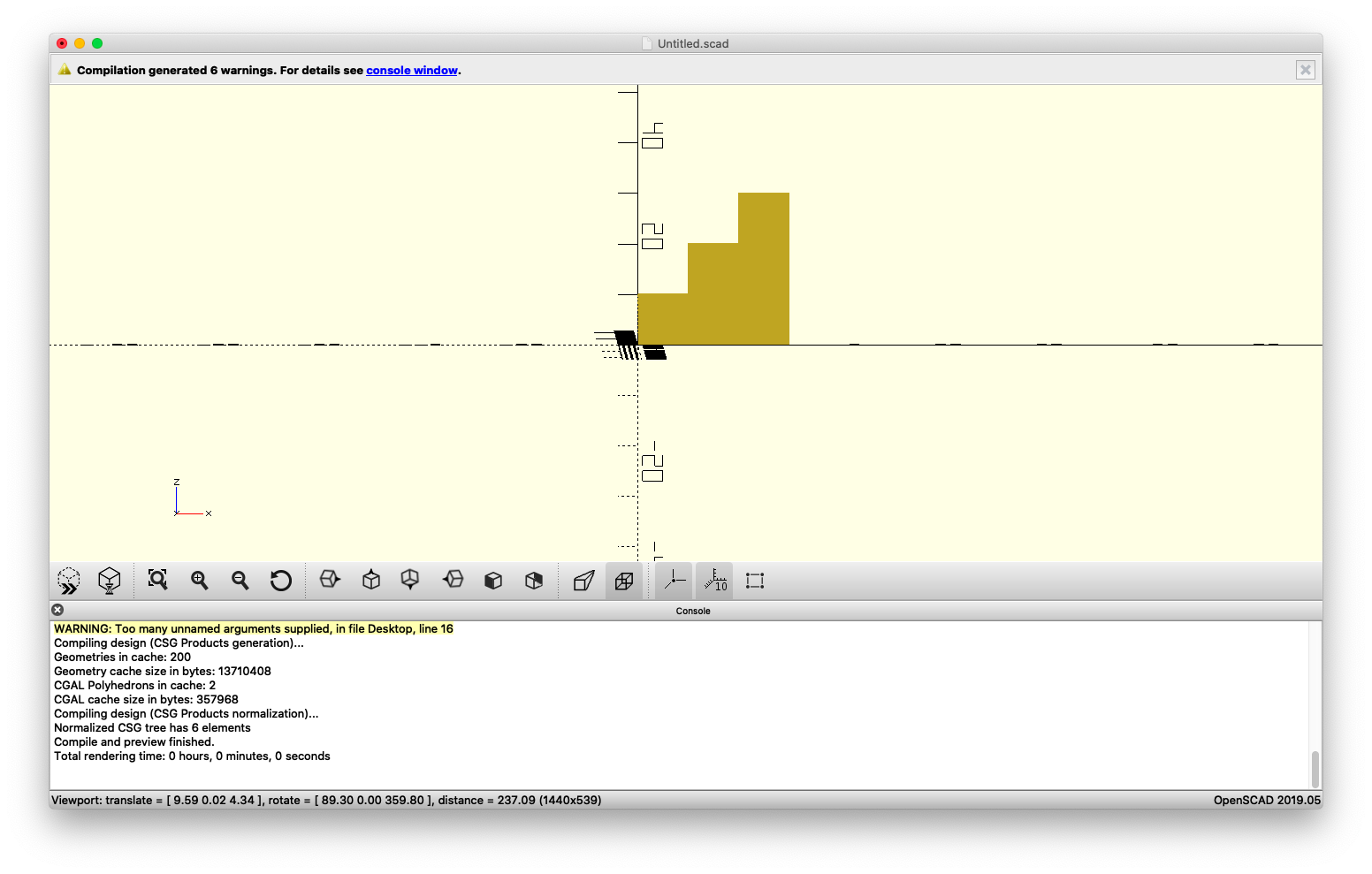Next, I got the bright idea to turn it into a flower. I soon found out exactly how much code that would require me to put in. But I eventually got this design, which to me just looks like a bunch of blocks stuck together.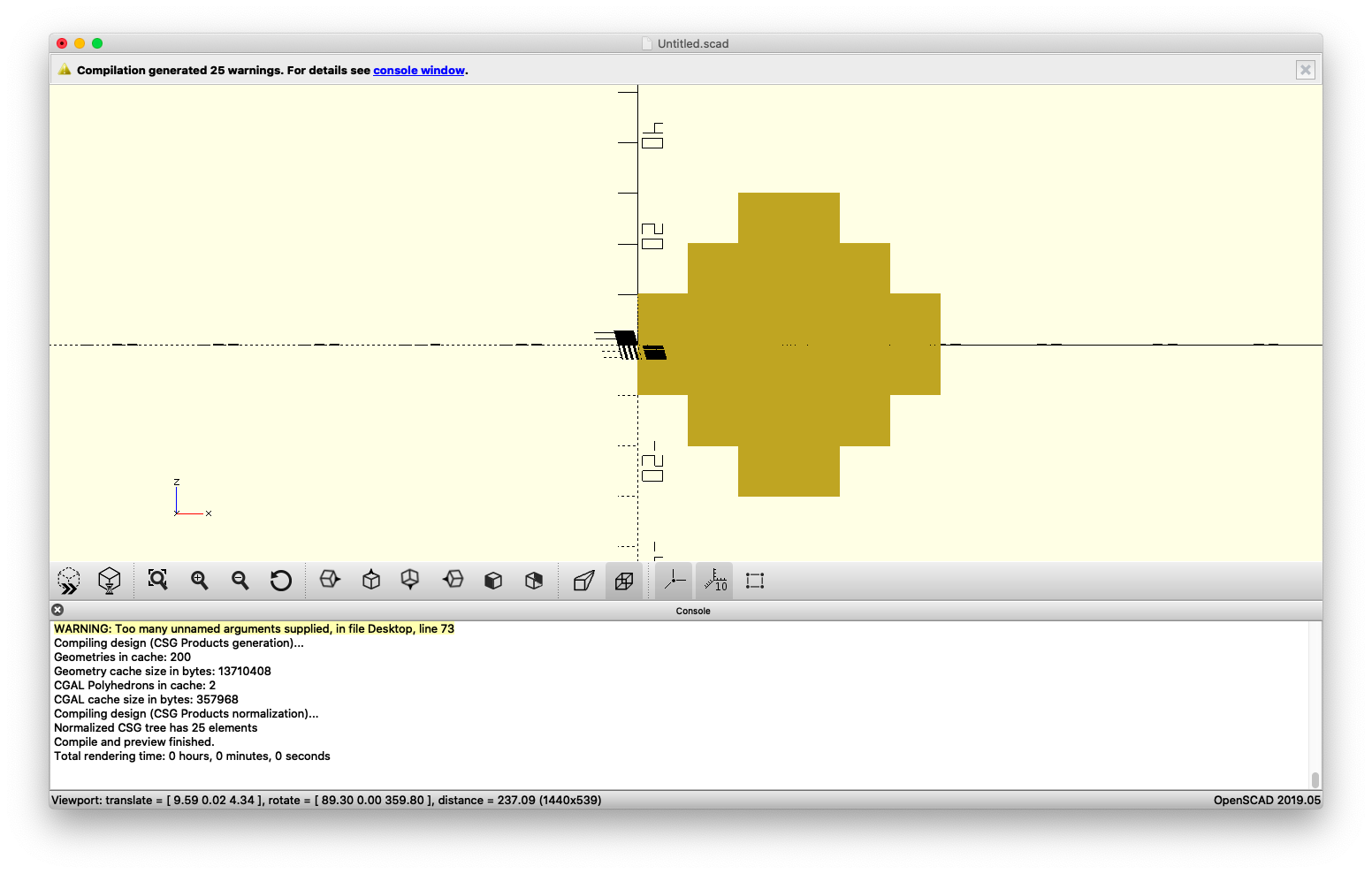Which meant I had to get even more creative and that is when I added in color and got this guy.(1980’s video games calling) In order to get this design I had to take out the center pieces and then re-add them as a separate code on the bottom. However, I still wasn’t happy with what I had so I decided to adjust the scaling.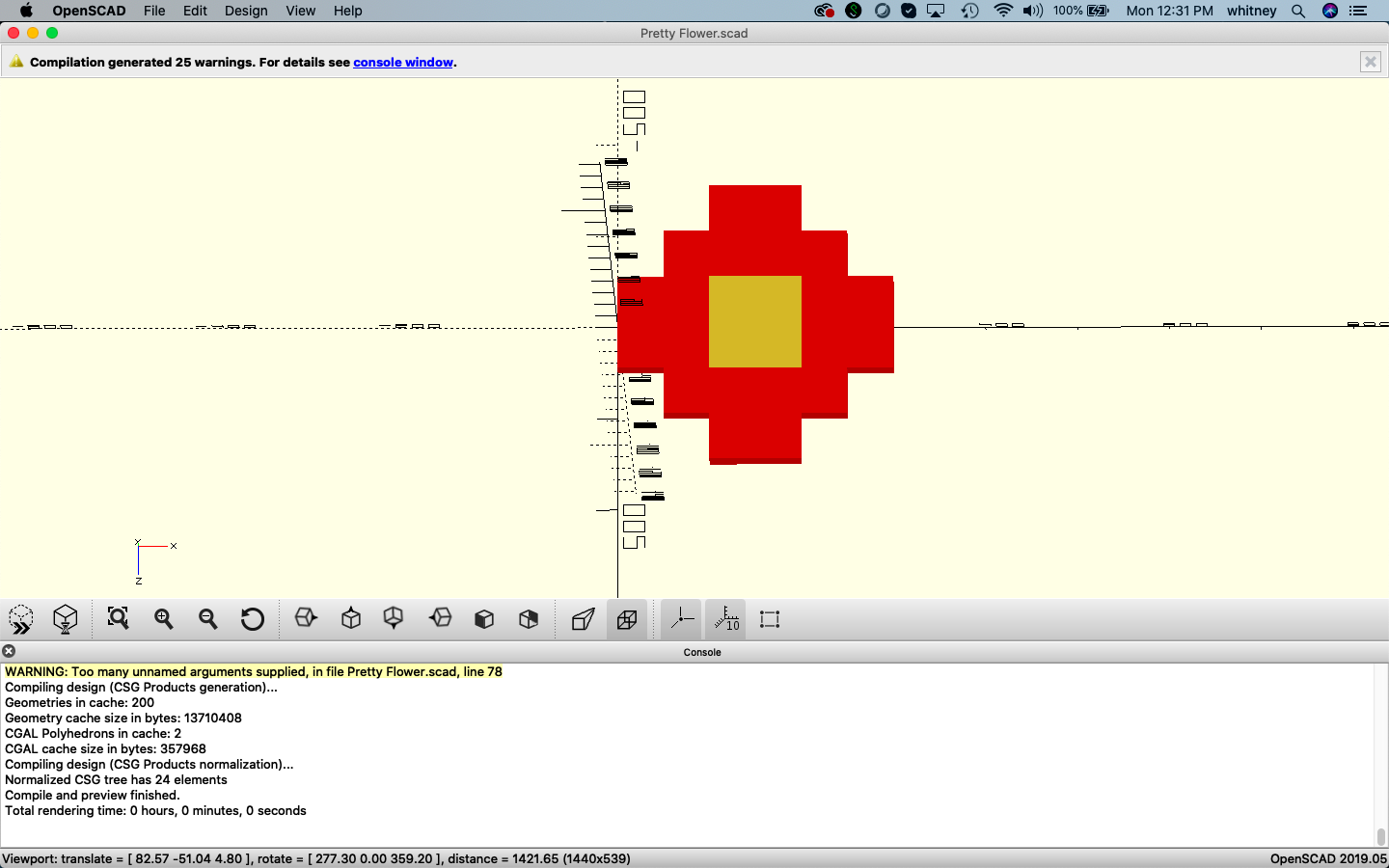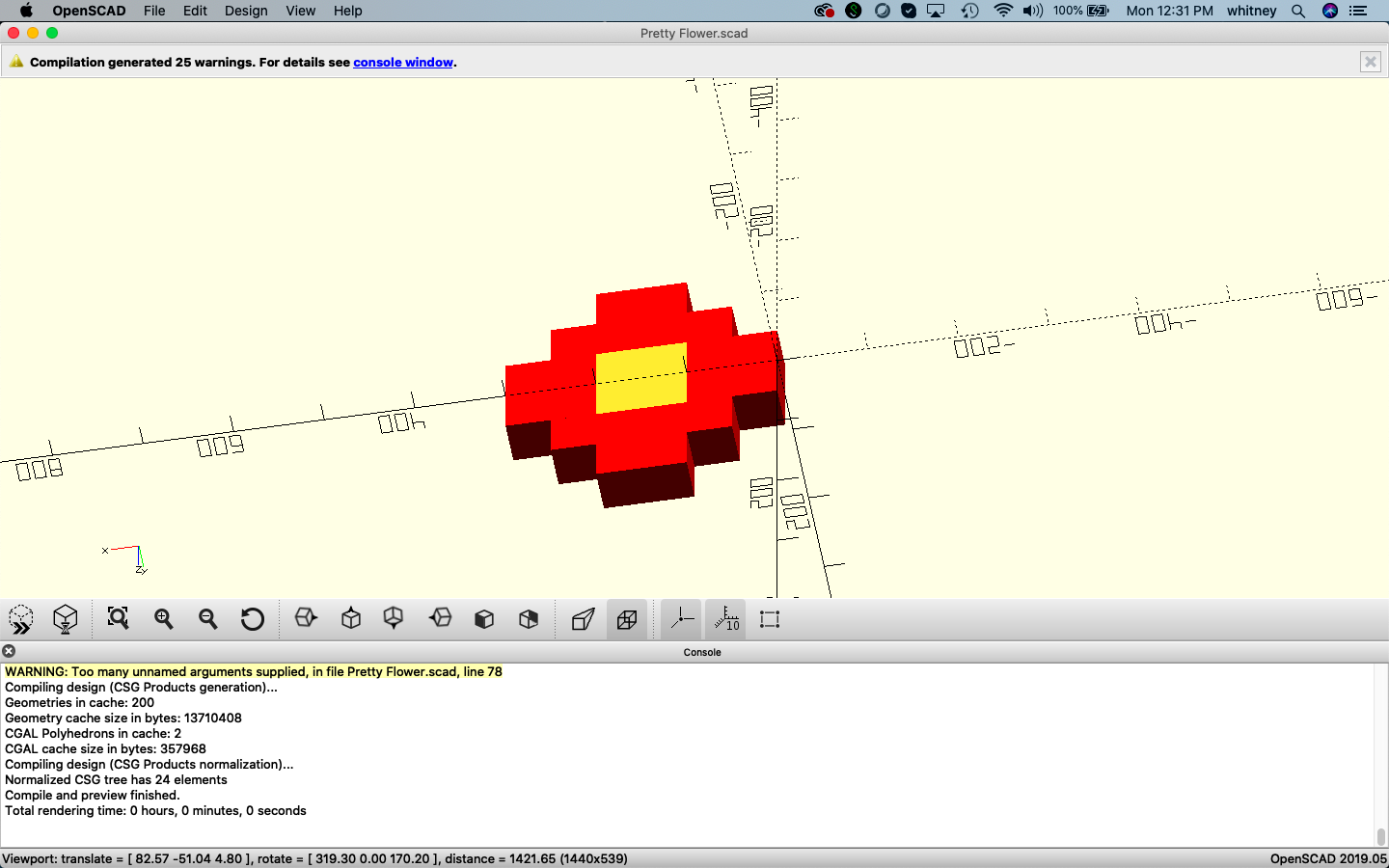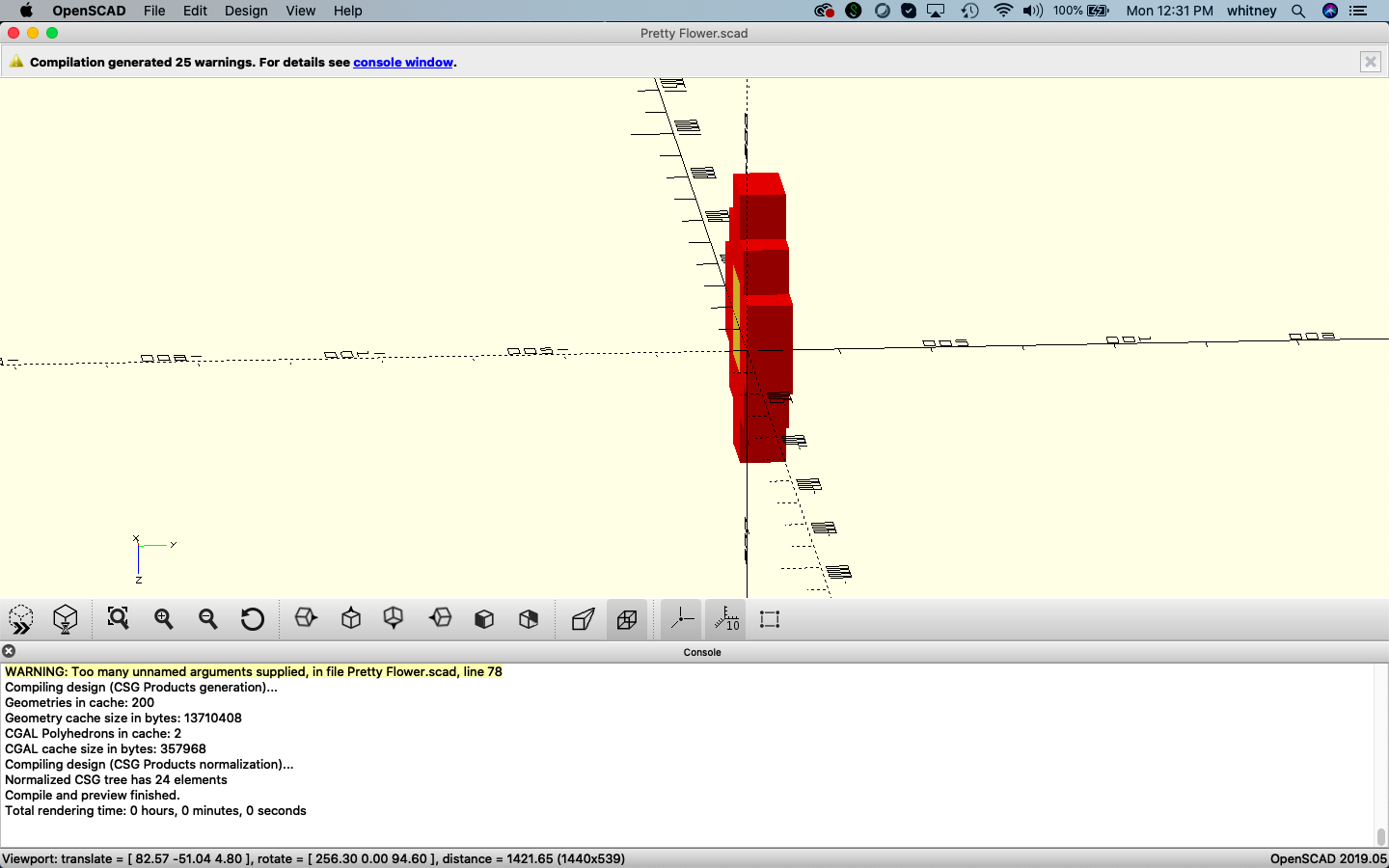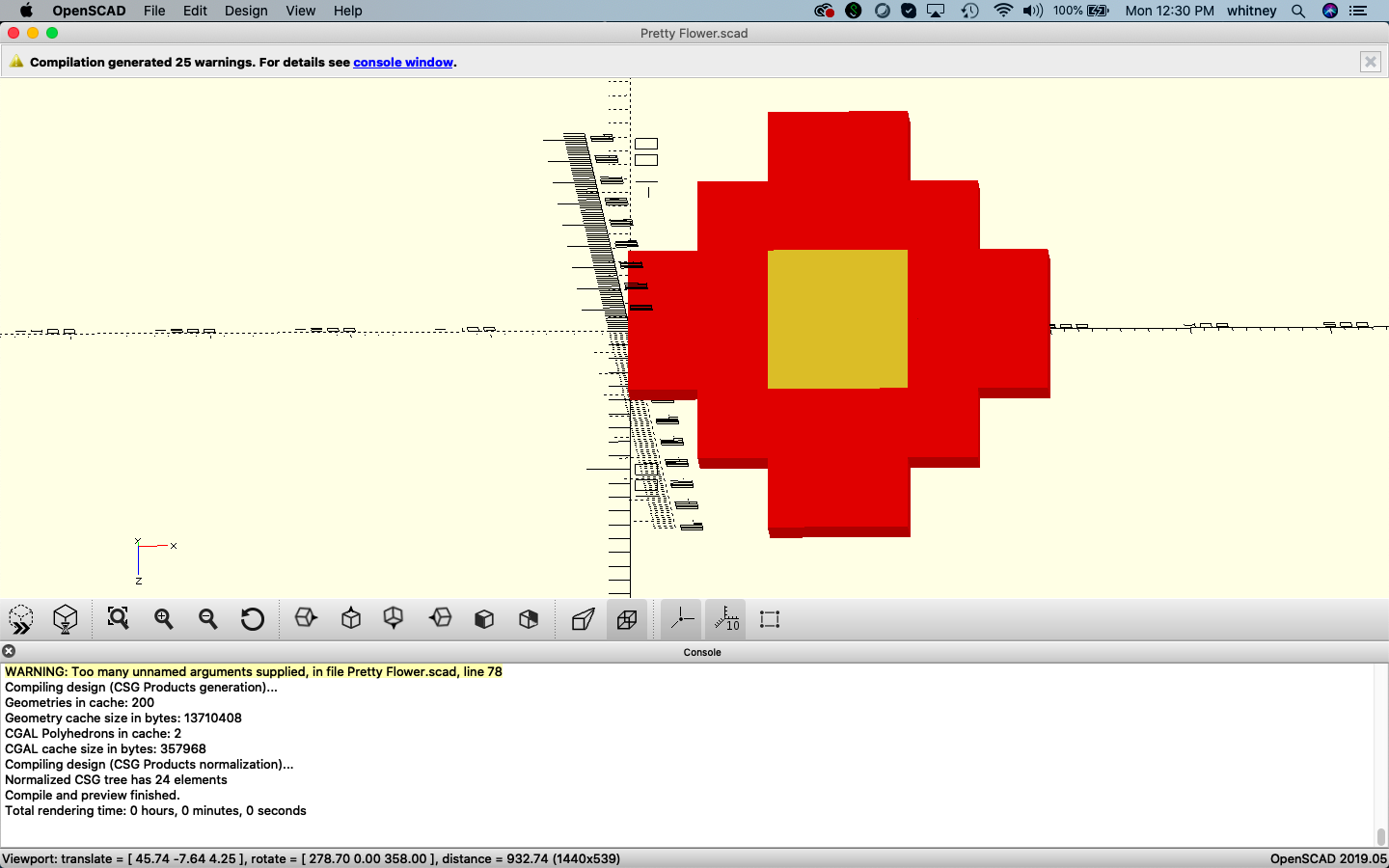Here’s the code I used to create my masterpiece. (For some reason I can’t get it to paste with the spacing)

```scale (5,5,5)
{

color ("red")
{

cube (10,10,10);

translate([10,0,0])
cube (10,10,10);

translate([10,0,10])
cube (10,10,10);

translate ([20,0,10])
cube  (10, 10, 10);

translate ([20,0,20])
cube (10,10,10);

translate ([30,0,20])
cube (10,10,10);

translate ([30,0,10])
cube (10,10,10);

translate ([40,0,10])
cube (10,10,10);

translate ([40,0,0])
cube (10,10,10);

translate ([50,0,0])
cube (10,10,10);

translate ([50,0,-10])
cube (10,10,10);

translate ([40,0,-10])
cube (10,10,10);

translate ([40,0,-20])
cube (10,10,10);

translate ([30,0,-20])
cube (10,10,10);

translate ([30,0,-30])
cube (10,10,10);

translate ([20,0,-30])
cube (10,10,10);

translate ([20,0,-20])
cube (10,10,10);

translate ([10,0,-20])
cube (10,10,10);

translate ([10,0,-10])
cube (10,10,10);

translate ([0,0,-10])
cube (10,10,10);

}

translate ([20,0,0])
cube (10,10,10);

translate ([20,0,-10])
cube (10,10,10);

translate ([30,0,0])
cube (10,10,10);

translate ([30,0,-10])
cube (10,10,10);

}```